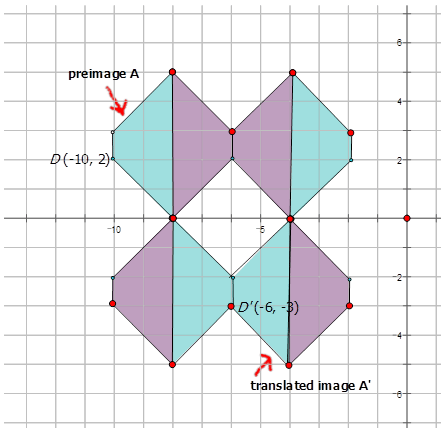# 8.9: Translation Notation

•• Contributed by CK12
• CK12

Graphical introduction to image translations. Learn to use notation to describe mapping rules,and graph images given preimage and translation

## Rules for Translations

Jack describes a translation as point moving from $$J(−2, 6)$$ to $$J′(4,9)$$. Write the mapping rule to describe this translation for Jack.

In geometry, a transformation is an operation that moves, flips, or changes a shape (called the preimage) to create a new shape (called the image). A translation is a type of transformation that moves each point in a figure the same distance in the same direction. Translations are often referred to as slides. You can describe a translation using words like "moved up 3 and over 5 to the left" or with notation. There are two types of notation to know.

1. One notation looks like $$T_{(3, 5)}$$. This notation tells you to add 3 to the $$x$$ values and add 5 to the $$y$$ values.
2. The second notation is a mapping rule of the form $$(x,y) \rightarrow (x−7, y+5)$$. This notation tells you that the $$x$$ and $$y$$ coordinates are translated to $$x−7$$ and $$y+5$$.

The mapping rule notation is the most common.

Interactive Element

Let's write a mapping rule for each of the following translations:

1. Sarah describes a translation as point $$P$$ moving from $$P(−2,2)$$ to $$P′(1,−1)$$.

In general, $$P(x, y) \rightarrow P′(x+a, y+b)$$.

In this case, $$P(−2, 2) \rightarrow P′(−2+a, 2+b)$$ or $$P(−2, 2) \rightarrow P′(1, −1)$$

Therefore:

$$\begin{array}{rr} -2+a=1 & \text { and } \quad 2+b=-1 \\ a=3 & \quad b=-3 \end{array}$$

The rule is: $$(x,y) \rightarrow (x+3, y−3)$$

1. Mikah describes a translation as point D in a diagram moving from $$D(1, −5)$$ to $$D′(−3,1)$$.

In general, $$P(x,y) \rightarrow P′(x+a, y+b)$$.

In this case, $$D(1,−5) \rightarrow D′(1+a,−5+b)$$ or $$D(1,−5) \rightarrow D′(−3,1)$$

Therefore:

$$\begin{array}{rrr} 1+a=-3 & \text { and } & -5+b=1 \\ a=-4 & & b=6 \end{array}$$

The rule is: $$(x,y) \rightarrow (x−4, y+6)$$

1. The translation of the preimage $$A$$ to the translated image $$J$$ in the diagram below:Figure $$\PageIndex{1}$$

First, pick a point in the diagram to use to see how it is translated.Figure $$\PageIndex{2}$$

$$D:(−1,4) D′:(6,1)$$

$$D(x,y) \rightarrow D′(x+a,y+b)$$

So: $$D(−1,4) \rightarrow D′(−1+a,4+b)$$ or $$D(−1,4) \rightarrow D′(6,1)$$

Therefore:

$$\begin{array}{rrr} -1+a=6 & \text { and } & 4+b=1 \\ a=7 & & b=-3 \end{array}$$

The rule is: $$(x,y) \rightarrow (x+7,y−3)$$

Example $$\PageIndex{1}$$

Earlier, you were told that Jack described a translation as point $$J$$ moving from $$J(−2,6)$$ to $$J′(4,9)$$. What is the mapping rule that describes this translation?

Solution

$$(x,y) \rightarrow (x+6, y+3)$$

Example $$\PageIndex{2}$$

Write the mapping rule that represents the translation of the red triangle to the translated green triangle in the diagram below.Figure $$\PageIndex{3}$$

Solution

$$(x, y) \rightarrow (x−3, y−5)$$

Example $$\PageIndex{3}$$

The following pattern is part of wallpaper found in a hotel lobby. Write the mapping rule that represents the translation of one blue trapezoid to a translated blue trapezoid shown in the diagram below.Figure $$\PageIndex{4}$$

Solution

If you look closely at the diagram below, there two pairs of trapezoids that are translations of each other. Therefore you can choose one blue trapezoid that is a translation of the other and pick a point to find out how much the shape has moved to get to the translated position.Figure $$\PageIndex{5}$$

For those two trapezoids: $$(x,y) \rightarrow (x+4, y−5)$$

## Review

Write the mapping rule to describe the movement of the points in each of the translations below.

1. $$S(1,5) \rightarrow S′(2,7)$$
2. $$W(−5,−1) \rightarrow W′(−3,1)$$
3. $$Q(2,−5) \rightarrow Q′(−6,3)$$
4. $$M(4,3) \rightarrow M′(−2,9)$$
5. $$B(−4,−2) \rightarrow B′(2,−2)$$
6. $$A(2,4) \rightarrow A′(2,6)$$
7. $$C(−5,−3) \rightarrow C′(−3,4)$$
8. $$D(4,−1) \rightarrow D′(−4,2)$$
9. $$Z(7,2) \rightarrow Z′(−3,6)$$
10. $$L(−3,−2) \rightarrow L′(4,−1)$$

Write the mapping rule that represents the translation of the preimage to the image for each diagram below.

1.Figure $$\PageIndex{6}$$
2.Figure $$\PageIndex{7}$$
3.Figure $$\PageIndex{8}$$
4.Figure $$\PageIndex{9}$$
5.Figure $$\PageIndex{10}$$